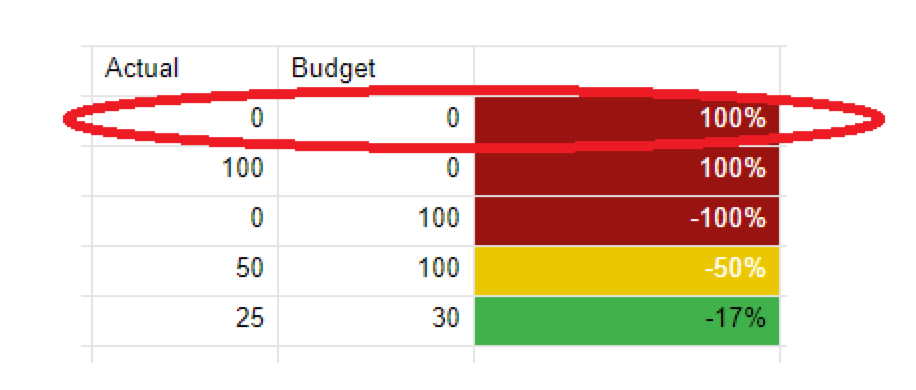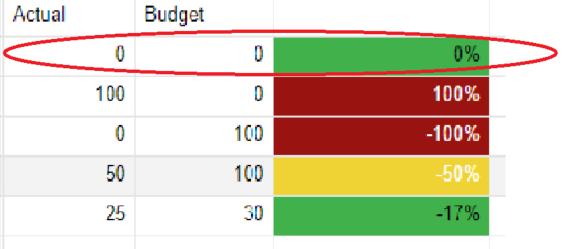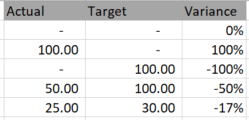#### Welcome to the Smartsheet Forum Archives

The posts in this forum are no longer monitored for accuracy and their content may no longer be current. If there's a discussion here that interests you and you'd like to find (or create) a more current version, please Visit the Current Forums.

# Calculating Variances with Negative Numbers

How do I do this on Smartsheet? I have an Actuals and a Budgets column. I'd like to compare the 2. So for example, if you have a Budget of 100, and an Actual of 70, then the variance would be -30%.

IFERROR((Actual-Target)/Target,0) is all good for positive numbers but I need to ensure it also works for negative numbers.

In Excel, this works using IFERROR((Actual-Target)/ABS(Actual*(Target=0)+Target),0).

How do I get a similar formula to work in Smartsheet?

Thanks

Tags:

• Elliot,

I must admit, I have never seen this:

ABS(Actual*(Target=0)+Target)

but I found a good link that explained it.

Excel formula returns variance for:

1. positive target (budget)

2. target of 0 (returns 100%)

3. negative target

4. returns 0 for other errors

Smartsheet does not have an easy way to prevent a Text/Number column from only being a number, so a typo could throw an error too.

This formula will account for that too (for row 23)

=IFERROR(IF(Target23 = 0, 1, (Actual23 - Target23) / ABS(Target23)), 0)

I hope this helps.

Craig

• Thanks v much Craig. That's super helpful.

• The power of the community.  Thanks Craig!

• Hi Craig,

It looks like the only scenario this formula does not work for is when your Actual and Budget are both 0.  Any suggestions?

Best Regards,

Marc• By my understanding of the Excel functionality, what you are seeing on row 1 (and 2) is case number 2: target (budget) of 0 returns 100%

=IFERROR(IF(Target23 = 0, 1, (Actual23 - Target23) / ABS(Target23)), 0)

I have bolded where this is determined. If you put any value in Actual (0, 20, 100, 1000), the Variance returned is 100%. Smarter people than I (and accountants too*) can debate what the variance should be when the budget / target is 0. The formula can then be changed to their opinion (the facts say 'undefined')

Craig

*that's a joke**

**mostly

• lol, Thanks Craig.  I get what you are saying and have seen a lot of debate on this.  For my purposes I need to show 0% change when Actual and Budget are both 0.  Basically what I am trying to do is highlight where the over and under spending is occurring across the budget. Is this even possible to do while keeping everything else in-line dynamically?  It does not appear to be.  Appreciate your help in advance.

In Excel this seems to work fine as I believe it should for my purposes.

Best Regards,

Marc• This formula will do that that for you:

=IFERROR(IF(AND(Target23 = 0, Actual23 = 0), 0, IF(Target23 = 0, 1, (Actual23 - Target23) / ABS(Target23))), 0)

However, if the Target is 0% and the the Actual is 100%, in some cases, that leads me to believe it can be something more than 0% and less than 100%. This will result (as before) with 100%

I would add some checks for that (conditional formatting or additional columns to check). Remember 0% Variance is GOOD and 100% is BAD, so I would avoid changing the formula for non-0 actuals when there was no budget.

Craig

• Thank you Craig this is perfect!

This discussion has been closed.